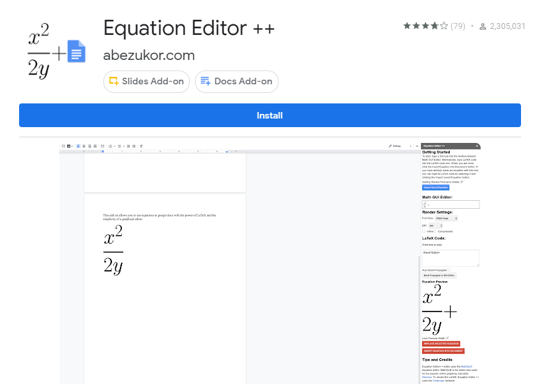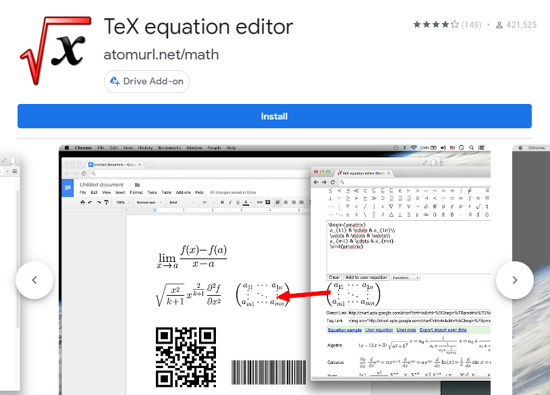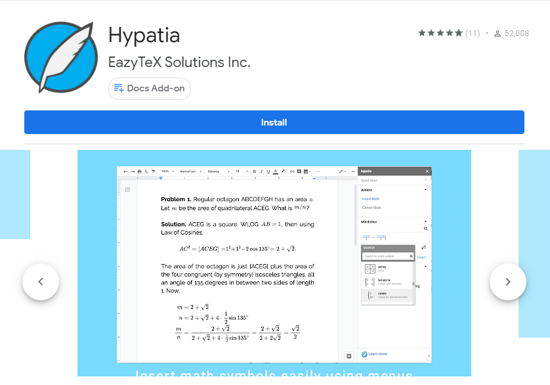Editor Ratings:
User Ratings:
[Total: 2 Average: 3.5]

When writing a scientific document in Google Docs, people are bound to face some challenges. These challenges include not able to write sentences with special characters or lengthy scientific equations. Moreover, there are people who work online most of the time but restrict themselves to writing a document in offline mode simply because the offline document provides the flexibility to insert the special characters and equations.

However, with news tools rolling out every day, these problems are easy to tackle. You can now pretty much write whatever you want in whatever format with using the right tools. Some of these tools are mentioned here in this document that lets you write the scientific calculations and math equation while you’re working in Google Docs online.

Here is the list of 5 Google Add one that you can use if you struggle to include math equations in the Google Docs. These add-ons are completely free and easily editable. Moreover, you can pretty much add some of them directly to your Google Docs Add-on panel.

### Equation Editor ++

Note: Using the Chrome browser to insert the equation in the Google Doc using this add-on works smoothly.### Auto-LaTex Equations

This add-on is a pretty advanced one if you want to beautify the equations in your document. Auto-LaTex lets you convert any math or scientific equation in the document into LaTex rendered images automatically. You simply have to write the math equations as you normally would do in the document and then open the add-on panel in the Google doc and open Auto-LaTex Equations. Enclose the equation in the document within \$\$……\$\$ and then simply click on the sidebar. After some time, all the equations will be renders in the LaTex format.

You can also adjust the equations by changing the font size, colors, and the merging of the equations to match the rest of the document. Also, if you did any mistake while rendering the equations, you can also de-render it using this add-on. However, you can only use it with Google Docs and not with other Google applications.

Try this add-on here### TeX Equation Editor

This particular add-on makes it simpler for the writer to write the mathematics equations and other scientific expressions in their Google Docs. This add-on helps you generate the image of mathematical equations, expression, special characters, and other operations easily. Not only the TeX equations, but you can also generate QR codes, Barcodes, and the other crypto codes in your Google Document.

This is a Google Drive Add-on, hence, you can use it pretty much with any document and paste the equations there.### MathType

MathType is an equation editor that helps you type in the equations in the Google Docs. To access this feature, you simply have to install this add-on and navigate to Add-on in an opened Google document. Then, you can click on “Math Type Beta”, where you will find the option of inserting or editing the math equation. Clicking that option will open up a window in which you can edit or insert the required equations using different tools, and then the same will be rendered into the document after you click on inserting it in the document. Moreover, this add-on is compatible with handwriting recognition using touch screens.

During the Coronavirus Pandemic, Mathtype is available for a free subscription for educational institutions until August 2020.### Hypatia

This one is among the best add-ons to get if you’re looking for a seamless way to insert and edit the math equations in Google docs. Hypatia is a free add-on that helps you insert and edit the equations in a fast and easy way. This math equation editor is supported by latex notation.

Install this add-on and access it simply by clicking on add-on in the Google Docs. Then, click on Hypatia to insert and edit the equations in a pop-window which will be updated in the Google docs almost instantly. This add-on is the effortless way to edit the equations as the formatting style of the equations will be in line with whole of the document. You can also quickly edit the equations from the sidebar panel.That’s all. These are the most suitable math equation editor by which you can edit and insert the math equations in Google Docs.

In brief

All these add-ons rely on the LaTex technology which pretty much helps you insert the Mathematical equations and other special scientific expressions in the Google Docs easily. Along with the math equations, some of these add-ons also helps you add the QR codes, Bar Codes, and other types of crypto codes in the document in a seamless manner. These types of math equation editors require some permission to edit the document and hence it is suggested to test and know all the requirements of the add-on so that the document privacy remains protected.

All these add-ons mentioned in this article are well curated in the privacy front as well, and you can use them pretty much with any document.

 Editor Ratings: User Ratings:[Total: 2 Average: 3.5] Free/Paid: Free Tags: math equation